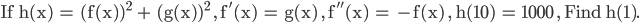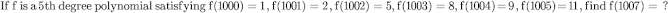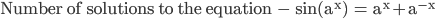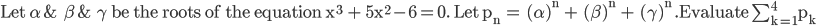Online Junior Mathematician Search
FOCUS AND ANSWER THE QUESTIONS CAREFULLY AS THE CUT-OFF VARIES. WRITE THE ANSWERS ONLY BELOW THE HEADING "ANSWER FOR QUESTION .. " .

EACH QUESTION IS WORTH 5 POINTS.

Name *
Email *
Mobile No. (In case we contact you)
Question 1 : a,b,c denote the lengths of a triangle & A,B,C the angles opposite to the sides.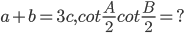Question 2 :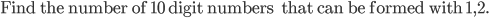Question 3 :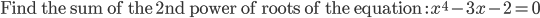Question 4 :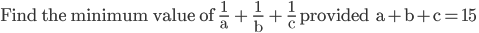Question 5 :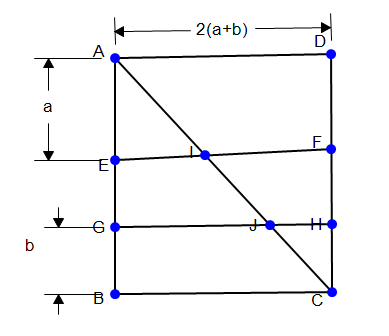The Given figure is a square ABCD of side length 2(a+b) units. AE = a units, BG = b units. They cut the diagonal AC at 'I' & 'J' respectively. Let the areas of AIFD,EIJG & JHC be X,Y,Z sq. units respectively. If Y = 2016 , Find X+Z ?
Question 6 : Find a 3-digit number which decreases 25 times when it's first digit is deleted ?
Question 7 :